This book is archived and will be removed July 6, 2022. Please use the updated version.

Capacitance

# 51 Energy Stored in a Capacitor

### Learning Objectives

By the end of this section, you will be able to:

• Explain how energy is stored in a capacitor
• Use energy relations to determine the energy stored in a capacitor network

Most of us have seen dramatizations of medical personnel using a defibrillator to pass an electrical current through a patient’s heart to get it to beat normally. Often realistic in detail, the person applying the shock directs another person to “make it 400 joules this time.” The energy delivered by the defibrillator is stored in a capacitor and can be adjusted to fit the situation. SI units of joules are often employed. Less dramatic is the use of capacitors in microelectronics to supply energy when batteries are charged ((Figure)). Capacitors are also used to supply energy for flash lamps on cameras.

The capacitors on the circuit board for an electronic device follow a labeling convention that identifies each one with a code that begins with the letter “C.” (credit: Windell Oskay)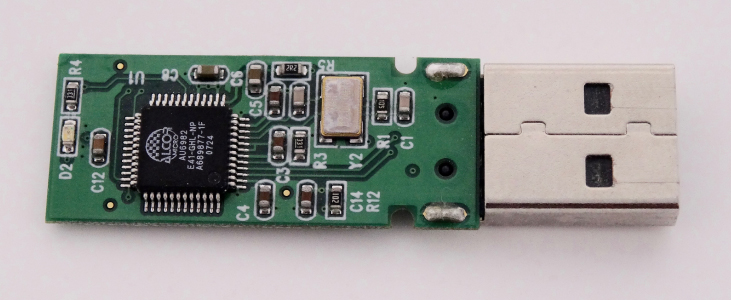The energy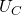stored in a capacitor is electrostatic potential energy and is thus related to the charge Q and voltage V between the capacitor plates. A charged capacitor stores energy in the electrical field between its plates. As the capacitor is being charged, the electrical field builds up. When a charged capacitor is disconnected from a battery, its energy remains in the field in the space between its plates.

To gain insight into how this energy may be expressed (in terms of Q and V), consider a charged, empty, parallel-plate capacitor; that is, a capacitor without a dielectric but with a vacuum between its plates. The space between its plates has a volume Ad, and it is filled with a uniform electrostatic field E. The total energyof the capacitor is contained within this space. The energy density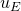in this space is simplydivided by the volume Ad. If we know the energy density, the energy can be found as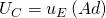. We will learn in Electromagnetic Waves (after completing the study of Maxwell’s equations) that the energy densityin a region of free space occupied by an electrical field E depends only on the magnitude of the field and is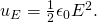If we multiply the energy density by the volume between the plates, we obtain the amount of energy stored between the plates of a parallel-plate capacitor: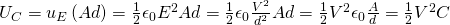.

In this derivation, we used the fact that the electrical field between the plates is uniform so that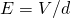and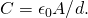Because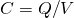, we can express this result in other equivalent forms: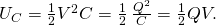The expression in (Figure) for the energy stored in a parallel-plate capacitor is generally valid for all types of capacitors. To see this, consider any uncharged capacitor (not necessarily a parallel-plate type). At some instant, we connect it across a battery, giving it a potential difference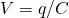between its plates. Initially, the charge on the plates is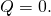As the capacitor is being charged, the charge gradually builds up on its plates, and after some time, it reaches the value Q. To move an infinitesimal charge dq from the negative plate to the positive plate (from a lower to a higher potential), the amount of work dW that must be done on dq is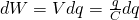.

This work becomes the energy stored in the electrical field of the capacitor. In order to charge the capacitor to a charge Q, the total work required is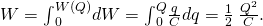Since the geometry of the capacitor has not been specified, this equation holds for any type of capacitor. The total work W needed to charge a capacitor is the electrical potential energystored in it, or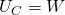. When the charge is expressed in coulombs, potential is expressed in volts, and the capacitance is expressed in farads, this relation gives the energy in joules.

Knowing that the energy stored in a capacitor is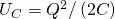, we can now find the energy densitystored in a vacuum between the plates of a charged parallel-plate capacitor. We just have to divideby the volume Ad of space between its plates and take into account that for a parallel-plate capacitor, we have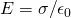and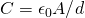. Therefore, we obtain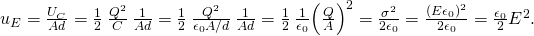We see that this expression for the density of energy stored in a parallel-plate capacitor is in accordance with the general relation expressed in (Figure). We could repeat this calculation for either a spherical capacitor or a cylindrical capacitor—or other capacitors—and in all cases, we would end up with the general relation given by (Figure).

Energy Stored in a Capacitor Calculate the energy stored in the capacitor network in (Figure)(a) when the capacitors are fully charged and when the capacitances are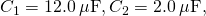and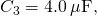respectively.

Strategy We use (Figure) to find the energy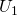,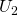, and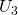stored in capacitors 1, 2, and 3, respectively. The total energy is the sum of all these energies.

Solution We identify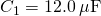and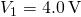,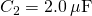and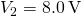,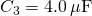and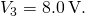The energies stored in these capacitors are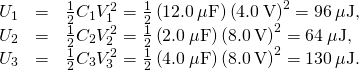The total energy stored in this network is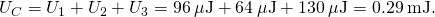Significance We can verify this result by calculating the energy stored in the single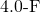capacitor, which is found to be equivalent to the entire network. The voltage across the network is 12.0 V. The total energy obtained in this way agrees with our previously obtained result,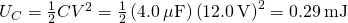.

Check Your Understanding The potential difference across a 5.0-pF capacitor is 0.40 V. (a) What is the energy stored in this capacitor? (b) The potential difference is now increased to 1.20 V. By what factor is the stored energy increased?

a.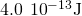; b. 9 times

In a cardiac emergency, a portable electronic device known as an automated external defibrillator (AED) can be a lifesaver. A defibrillator ((Figure)) delivers a large charge in a short burst, or a shock, to a person’s heart to correct abnormal heart rhythm (an arrhythmia). A heart attack can arise from the onset of fast, irregular beating of the heart—called cardiac or ventricular fibrillation. Applying a large shock of electrical energy can terminate the arrhythmia and allow the body’s natural pacemaker to resume its normal rhythm. Today, it is common for ambulances to carry AEDs. AEDs are also found in many public places. These are designed to be used by lay persons. The device automatically diagnoses the patient’s heart rhythm and then applies the shock with appropriate energy and waveform. CPR (cardiopulmonary resuscitation) is recommended in many cases before using a defibrillator.

Automated external defibrillators are found in many public places. These portable units provide verbal instructions for use in the important first few minutes for a person suffering a cardiac attack. (credit: Owain Davies)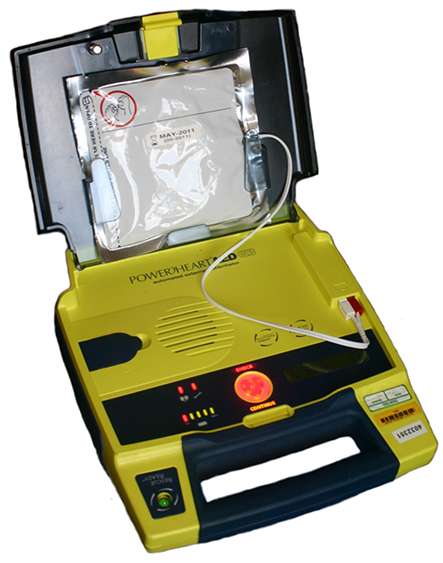Capacitance of a Heart Defibrillator A heart defibrillator delivers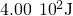of energy by discharging a capacitor initially at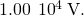What is its capacitance?

Strategy We are givenand V, and we are asked to find the capacitance C. We solve (Figure) for C and substitute.

Solution Solving this expression for C and entering the given values yields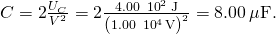### Summary

• Capacitors are used to supply energy to a variety of devices, including defibrillators, microelectronics such as calculators, and flash lamps.
• The energy stored in a capacitor is the work required to charge the capacitor, beginning with no charge on its plates. The energy is stored in the electrical field in the space between the capacitor plates. It depends on the amount of electrical charge on the plates and on the potential difference between the plates.
• The energy stored in a capacitor network is the sum of the energies stored on individual capacitors in the network. It can be computed as the energy stored in the equivalent capacitor of the network.

### Conceptual Questions

If you wish to store a large amount of energy in a capacitor bank, would you connect capacitors in series or parallel? Explain.

### Problems

How much energy is stored in an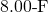capacitor whose plates are at a potential difference of 6.00 V?

A capacitor has a charge of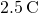when connected to a 6.0-V battery. How much energy is stored in this capacitor?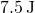How much energy is stored in the electrical field of a metal sphere of radius 2.0 m that is kept at a 10.0-V potential?

(a) What is the energy stored in the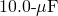capacitor of a heart defibrillator charged to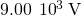? (b) Find the amount of the stored charge.

a. 405 J; b. 90.0 mC

In open-heart surgery, a much smaller amount of energy will defibrillate the heart. (a) What voltage is applied to the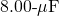capacitor of a heart defibrillator that stores 40.0 J of energy? (b) Find the amount of the stored charge.

A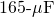capacitor is used in conjunction with a dc motor. How much energy is stored in it when 119 V is applied?

1.17 J

Suppose you have a 9.00-V battery, a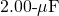capacitor, and a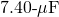capacitor. (a) Find the charge and energy stored if the capacitors are connected to the battery in series. (b) Do the same for a parallel connection.

An anxious physicist worries that the two metal shelves of a wood frame bookcase might obtain a high voltage if charged by static electricity, perhaps produced by friction. (a) What is the capacitance of the empty shelves if they have area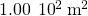and are 0.200 m apart? (b) What is the voltage between them if opposite charges of magnitude 2.00 nC are placed on them? (c) To show that this voltage poses a small hazard, calculate the energy stored. (d) The actual shelves have an area 100 times smaller than these hypothetical shelves. Are his fears justified?

a.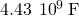; b. 0.453 V; c.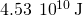; d. no

A parallel-plate capacitor is made of two square plates 25 cm on a side and 1.0 mm apart. The capacitor is connected to a 50.0-V battery. With the battery still connected, the plates are pulled apart to a separation of 2.00 mm. What are the energies stored in the capacitor before and after the plates are pulled farther apart? Why does the energy decrease even though work is done in separating the plates?

Suppose that the capacitance of a variable capacitor can be manually changed from 100 pF to 800 pF by turning a dial, connected to one set of plates by a shaft, from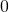to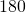. With the dial set at(corresponding to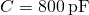), the capacitor is connected to a 500-V source. After charging, the capacitor is disconnected from the source, and the dial is turned to. If friction is negligible, how much work is required to turn the dial fromto?

0.7 mJ

### Glossary

energy density
energy stored in a capacitor divided by the volume between the plates